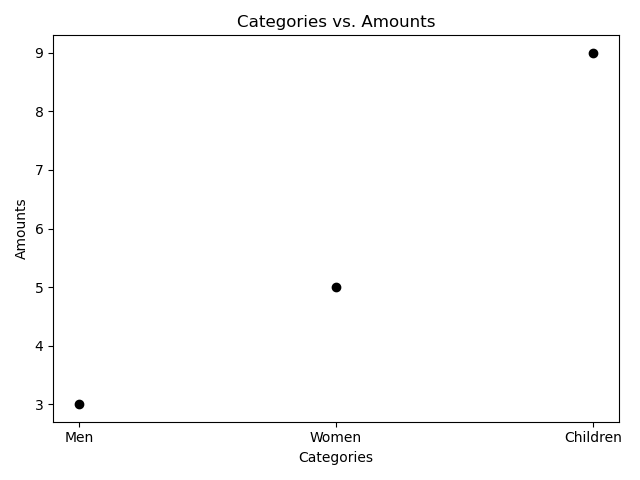# # How to draw a line graph with matplotlib

To draw any graph or chart, we need to first create a `Figure`.

Inside a figure, we can draw graphs or charts.

In matplotlib, graphs or charts are called plots, and plots are drawn on top of axes (the plural for axis). Most plots are drawn on x and y axes, although some plots can be drawn on 3-dimensional axes as well.

The code below creates a figure with `plt.figure()`, plots data points using `plt.plot()`, and then displays the resultant graph (by default, by bringing up a window on your screen).

``````import matplotlib.pyplot as plt

plt.figure()
plt.plot([1, 2, 3, 4], [3, 5, 9, 25])
plt.show()
``````

## # Plotting data

`plt.plot()` is used to plot any arbitrary data. It automatically creates a set of 2D axes for you, and by default it produces a line graph: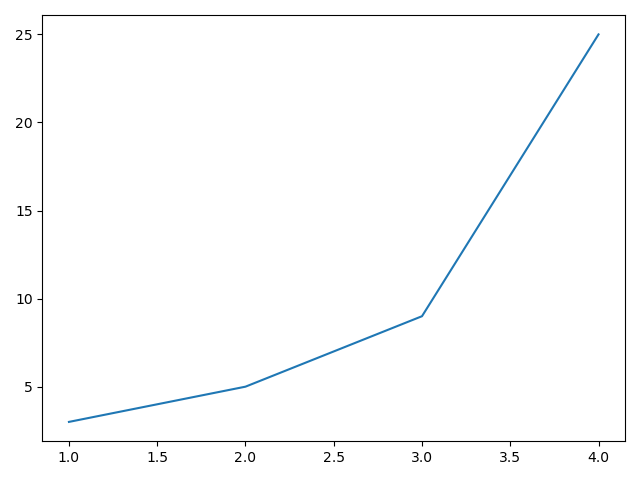This is achieved by joining the points plotted with a straight line.

Note the first argument to `.plot()` is the x-axis values, and the second argument is the y-axis values.

But it can be changed, for example to draw circles:

``````import matplotlib.pyplot as plt

plt.figure()
plt.plot([1, 2, 3, 4], [3, 5, 9, 25], 'o')
plt.show()
``````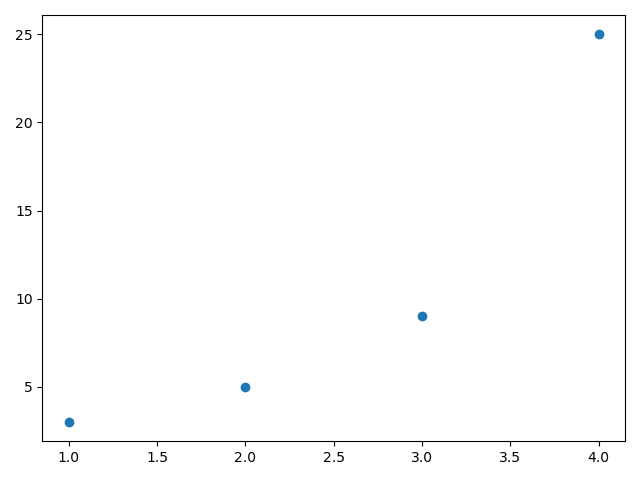## # Using built-in colours

You can pass in a colour from the list of built-in colours[1:1] as part of the string that defines the shape of the points:

``````import matplotlib.pyplot as plt

plt.figure()
plt.plot([1, 2, 3, 4], [3, 5, 9, 25], 'ko')
plt.show()
``````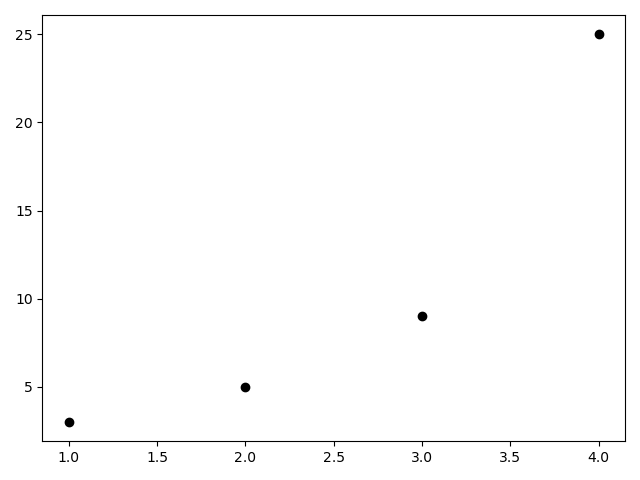### # Using custom colours

You can use custom colours by passing the colour argument separately:

``````import matplotlib.pyplot as plt

plt.figure()
lines = plt.plot([1, 2, 3, 4], [3, 5, 9, 25])
plt.setp(lines, color="#ff5566")
plt.show()
``````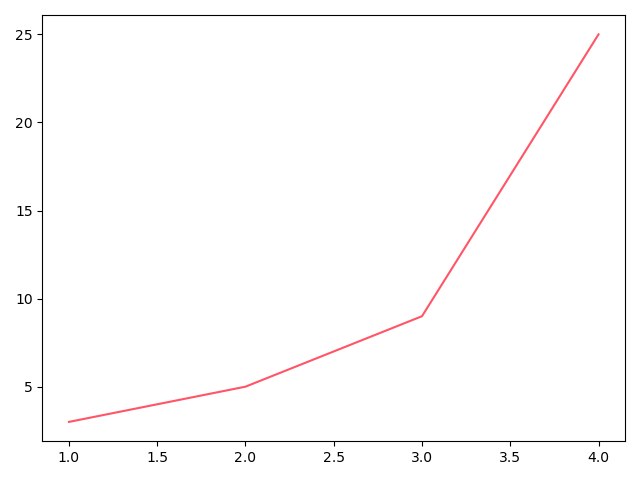## # Categorical variables

Instead of numbers for the x axis, you can pass in names to be used as categories:

``````import matplotlib.pyplot as plt

plt.figure()
plt.plot(["Men", "Women", "Children"], [3, 5, 9], 'ko')
plt.show()
``````

## # Adding axis labels and title

We can also add axis labels with `plt.xlabel("Categories")` and `plt.ylabel("Amounts")`, for example.

A title for the graph can be added with `plt.title("Categories vs. Amounts")`.

``````import matplotlib.pyplot as plt

plt.figure()

plt.xlabel("Categories")
plt.ylabel("Amounts")
plt.title("Categories vs. Amounts")

plt.plot(["Men", "Women", "Children"], [3, 5, 9], 'ko')
plt.show()
``````